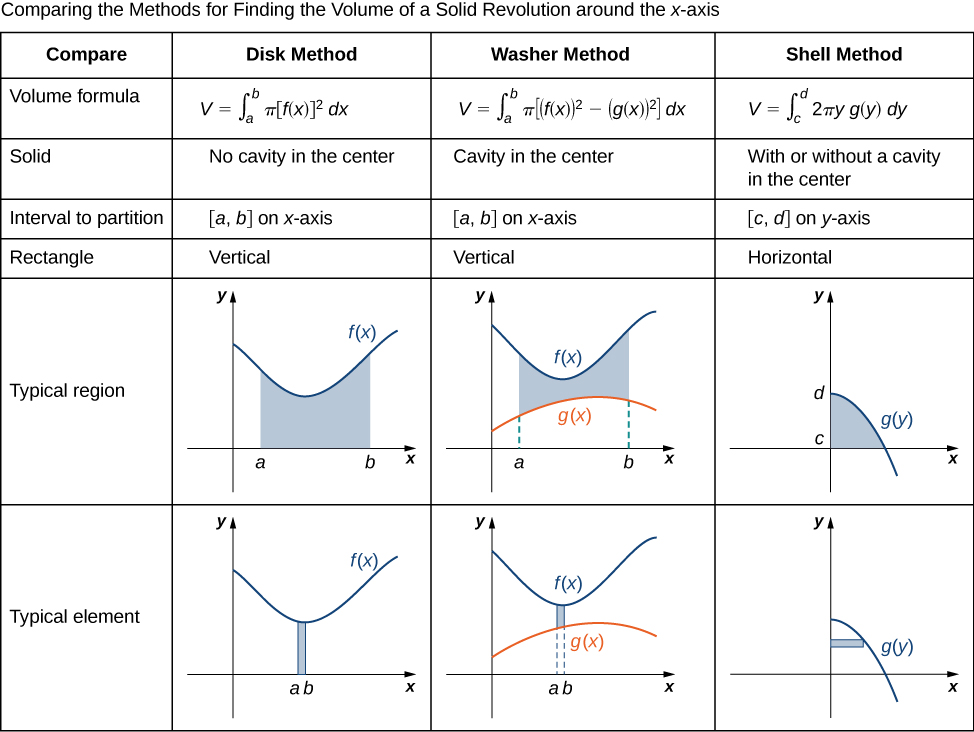## Comparing Methods for Volume Calculation

### Learning Outcomes

• Compare the different methods for calculating a volume of revolution

We have studied several methods for finding the volume of a solid of revolution, but how do we know which method to use? It often comes down to a choice of which integral is easiest to evaluate. Figure 10 describes the different approaches for solids of revolution around the $x\text{-axis}.$ It’s up to you to develop the analogous table for solids of revolution around the $y\text{-axis}.$Figure 10.

Let’s take a look at a couple of additional problems and decide on the best approach to take for solving them. Read the tips below that can help you decide the best method:

 x-axis revolution y-axis revolution Disk/washer: $dx$ Shell: $dy$ Disk/washer: $dy$ Shell: $dx$

Most times, functions are presented in terms of $x$ so if possible, keeping things in terms of $x$ is beneficial. This means that generally speaking, for an $x$-axis revolution, a disk/washer method will allow us not to have to rewrite the equation in terms of $y$ and for a $y$-axis revolution, the shell method will allow us the same advantage.

Also, keep in mind that for the disk/washer method, your rectangles will always be perpendicular to your axis of revolution. For the shell method, your rectangles will be parallel to your axis of revolution.

Using this information, try to draw your rectangles in terms of $dx$, if possible. If this requires you to separate the area, try the $dy$ method!

### Example: Selecting the Best Method

For each of the following problems, select the best method to find the volume of a solid of revolution generated by revolving the given region around the $x\text{-axis},$ and set up the integral to find the volume (do not evaluate the integral).

1. The region bounded by the graphs of $y=x,$ $y=2-x,$ and the $x\text{-axis}.$
2. The region bounded by the graphs of $y=4x-{x}^{2}$ and the $x\text{-axis}.$

### Try It

Select the best method to find the volume of a solid of revolution generated by revolving the given region around the $x\text{-axis},$ and set up the integral to find the volume (do not evaluate the integral): the region bounded by the graphs of $y=2-{x}^{2}$ and $y={x}^{2}.$

Watch the following video to see the worked solution to Example: Selecting the Best Method and the above Try It.